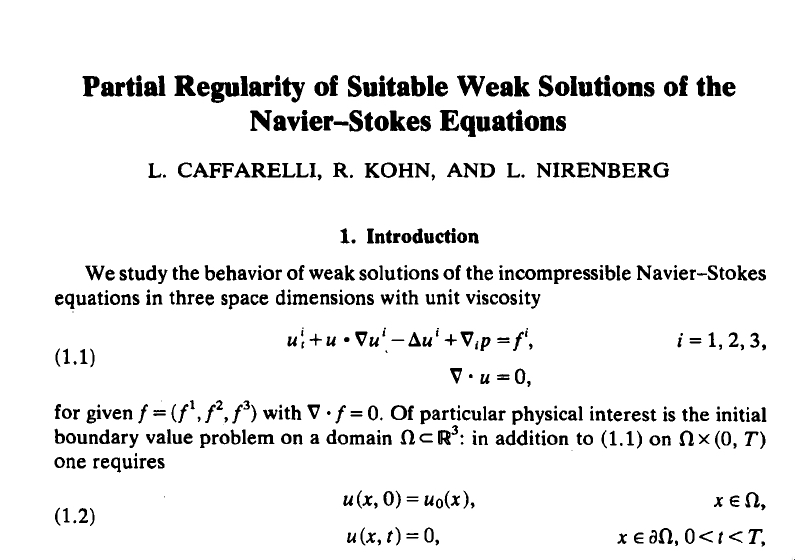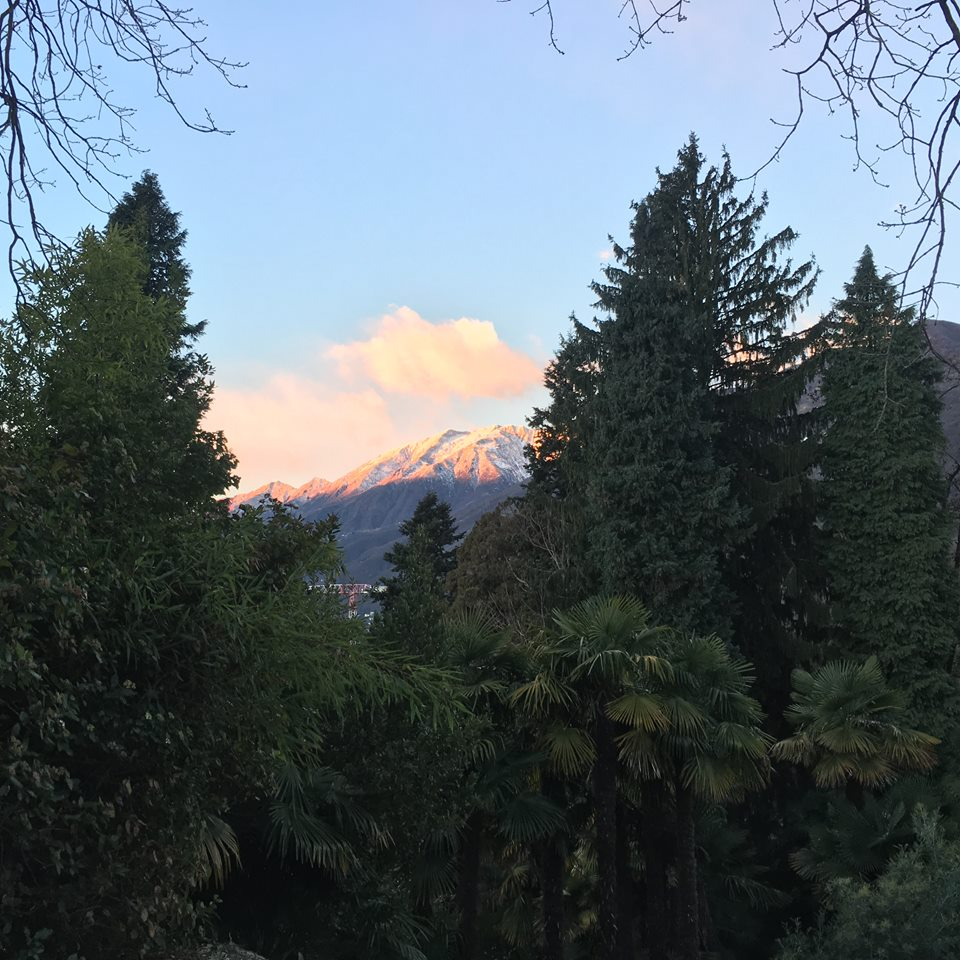# Nonlinear Schrödinger as a Dynamical System¶

## Overview of Lecture 4¶

• Review of the main issue: understand the dynamics.
• Critical Scattering Conjecture?
• Robust Methods for Global Well-posedness?
• Partial Regularity?
• High Regularity Globalizing Estimates?

# Initial Value Problem for NLS¶

$$\begin{equation*} \tag{NLS^{\pm}_p (\Omega)} \left\{ \begin{matrix} (i \partial_t + \Delta) u = \pm |u|^{p-1} u \\ u(0,x) = u_0 (x), ~ x \in \Omega. \end{matrix} \right. \end{equation*}$$

## Dilation Invariance¶

One solution $u$ generates parametrized family $\{u^\lambda\}_{\lambda > 0}$ of solutions:

$$u:[0,T) \times \mathbb{R}^d_x \rightarrow \mathbb{C} ~{\mbox{solves}}~ NLS_p^{\pm}(\mathbb{R}^d)$$

$${\iff}$$$$u^\lambda: [0,\lambda^2 T )\times \mathbb{R}^d_x \rightarrow \mathbb{C} ~{\mbox{solves}}~ NLS_p^{\pm}(\mathbb{R}^d)$$

where $$u^\lambda (\tau, y) = \lambda^{-2/(p-1)} u( \lambda^{-2} \tau, \lambda^{-1} y).$$

## Critical Regularity Regimes¶

critical Sobolev index Regime
$s_c < 0$ mass subcritical
$0 < s_c < 1$ mass super/energy subcritical
$s_c = 1$ energy critical
$1 < s_c < \frac{d}{2}$ energy supercritical

# Critical Scattering Conjecture?¶

$H^{s_c} ({\mathbb{R}}^d) \ni u_0 \longmapsto u$ solves defocusing $NLS_p^{\pm}(\mathbb{R}^d)$ globally in time with globally bounded spacetime Strichartz size.

$\implies$ the behavior of $u(t)$ is described by associated linear evolutions as $t \rightarrow \pm \infty$.

## Status of Critical Scattering?¶

• Energy Critical ($s_c = 1$): $\checkmark$
• Mass Critical ($s_c = 0$): $\checkmark$
• $0 < s_c < 1$: OPEN
• Energy Supercritical ($s_c > 1$): OPEN

## Remarks¶

• Conditional results under critical norm bounds $$\sup_t \| u(t) \|_{H^{s_c}} < \infty.$$
• Interesting analogies with global issue for Navier-Stokes.
• Corresponding problems for NLW might be resolved first.
• $H^{1/2}$-Critical seems most tractable, e.g. $NLS_3^+ ({\mathbb{R}}^3)$.

## Local to Global via Almost Conservation¶

The almost conservation property $$\sup_{t \in [0,T_{lwp}]} \widetilde{E} [I u(t)] \leq \widetilde{E}[Iu_0] + N^{-\alpha}$$ led to GWP for $$s > s_\alpha = \frac{2}{2+\alpha}.$$

## Better Bootstraps?¶

• Bootstrap arguments using Morawetz control generated improvements.
• Precise bootstraps with Raphaël led to results for $s > s_c$.
• Q: Could improved bootstraps lead to robust method to prove global regularity for $s>s_c$?

Addendum from discussion after the talk! See recent work by Ben Dodson on NLW. Recently, Dodson announced corresponding results for $NLS_3^+ (\mathbb{R}^3)$.

# Partial Regularity?¶## Notations for $NLS_p^+ (\mathbb{R}^d)$.¶

$$u_\lambda (t,x) = [\frac{1}{\lambda}]^{\frac{2}{p-1}} u(\frac{t}{\lambda^2}, \frac{x}{\lambda}).$$

Exponents appearing in dilation invariant spaces used in the study of $NLS_p$: $$s_c = \frac{d}{2} - \frac{2}{p-1}, ~\mbox{(Scaling invariant Sobolev index)}$$ $$\frac{2}{q} + \frac{d}{r} = \frac{2}{p-1}, ~(H^{s_c} ~\mbox{admissible Strichartz pairs} ~(q,r))$$ $$\frac{d}{p_c} = \frac{2}{p-1}, ~\mbox{(Scaling invariant spatial Lebesgue space exponent)}$$ $$\frac{2+d}{q_c} = \frac{2}{p-1}, ~\mbox{(Diagonal scaling invariant Strichartz exponent)}.$$

## The Singular Set¶

• Spacetime point $z_0 = (t_0, x_0) \in \mathbb{R} \times {\mathbb{R}}^d.$
• $Q_\lambda$ denote the parabolic box of scale $\lambda$ behind $z_0$ $$\{(t,x) \in \mathbb{R}^{1+d}: 0< t_0 - t < \lambda^2, |x - x_0| < \lambda\}.$$

Definition: A spacetime point $z_0$ is called a singular point for a weak solution $u$ of $NLS_p$ if $$\lim_{\lambda \searrow 0} \int_{Q_\lambda} |u|^{q_c} dx dt = + \infty.$$ Points which are not singular are called regular points. The set of all singular points is denoted $\Sigma$. The diagonal Strichartz norm diverges on all parabolic boxes behind a singular point. Points which are not singular are called regular points for $u$.

## Concentration Statement?¶

Singular Point $\implies$ Consistent $L^{q_c}_{t,x}$ Concentration: If $z_0$ is a singular point for the weak solution $u$ then $z_0$ is also a point of (scaling consistent) concentration. That is, there exists $\epsilon_0 > 0$ (a constant independent of $z_0$) and a sequence of scales $\lambda_j \searrow 0$ satisfying $$\int_{Q_{\lambda_j}} |P_{< \frac{1}{\lambda_j}} u|^{q_c} dx dt > \epsilon_0.$$

The converse would be an "$\epsilon$-regularity" statement.

## Parabolic Hausdorff Dimension of $\Sigma$?¶

Absorption of Interaction Morawetz $\implies {\mbox{dim}}_{\mathscr{P}} \Sigma \leq 4 (s_c - \frac{1}{4})$

## High Regularity Globalizing Estimates?¶

Consider the $H^2$-critical problem $$\begin{equation*} \tag{NLS^{\pm}_5 (\mathbb{R}^5)} \left\{ \begin{matrix} (i \partial_t + \Delta) u = \pm |u|^{4} u \\ u(0,x) = u_0 (x), ~ x \in \mathbb{R}^5. \end{matrix} \right. \end{equation*}$$

Assume $u_0$ is nice (smooth, compactly supported). Can one prove that $$\sup_t \| u(t) \|_{H^k (\mathbb{R}^5)} < \infty?$$

Possible ingredients: Almost conservation techniques, a priori spacetime estimates, Gronwall estimate, contradiction arguments, ...?

# Thank you!¶